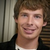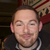+1

# Is it possible to alternate background colors on tableviews?

thillsman 8 years ago updated by icahill (Administrator) 8 years ago
Ok, tricky one for you (maybe). Look at the iOS 6 Music app. Navigate into an artist, then an album. All the songs are listed, and the backgrounds alternate by row. I want to do that on a tableview. Here's what I tried:

``` <class name="itemsclass"> <panel name="[param:index]" width="100%" height="70" align="left" _index="[param:index]" background="666666" padding="5" onclickup="showdetails" lhs="[eval:Math.pow(-1,[param:index])]" operator="e" rhs="1"> <text name="title" text="[param:title]" width="80%" align="center" valign="center" alignment="left" height="fit" font="Avenir" size="24" color="#333333" decode="yes" maxlength="65" endcap="..."></text> </panel> <panel name="[param:index]" width="100%" height="70" align="left" _index="[param:index]" background="333333" padding="5" onclickup="showdetails" lhs="[eval:Math.pow(-1,[param:index])]" operator="e" rhs="-1"> <text name="title" text="[param:title]" width="80%" align="center" valign="center" alignment="left" height="fit" font="Avenir" size="24" color="#333333" decode="yes" maxlength="65" endcap="..."></text> </panel> </class> ```

The key parts (I think) are the lhs/operator/rhs attributes. It's my understanding that using those attributes is basically and if statement that will create the panel (if true) or not (if false). The logic goes like this: if -1 to the Xth power (where X is the index) is equal to 1, it's an even numbered row and should be #666666 in color. If it is equal to -1, it's an odd numbered row and should be #333333 in color.

In theory, I think this makes sense. In reality, it's only showing one background color (though it shows all the items from the datasource). Any glaring mistakes or helpful tips?Tyler,

Here is a solution for you. If you use this example in your class for the list items.
On your class background you can add this to the alpha attribute:

alpha="[eval: [datasource:myDatasourceName.dataSourceIndex] % 2 == 0 ? 0 : 1 ]"

Modulus (%) is just about the remainder. If you want to know if a number is even or odd, modulus it by 2 and if there is a remainder the number is odd.This means you only need one class.If you put it directly in the class, you don't have to use the datasource:, you can just do something like this:

``` <panel name="background" background="#00ff00" height="100%" width="100%" alpha="[eval: [param:dataSourceIndex] % 2 == 0 ? 0 : 1 ]" /> ```